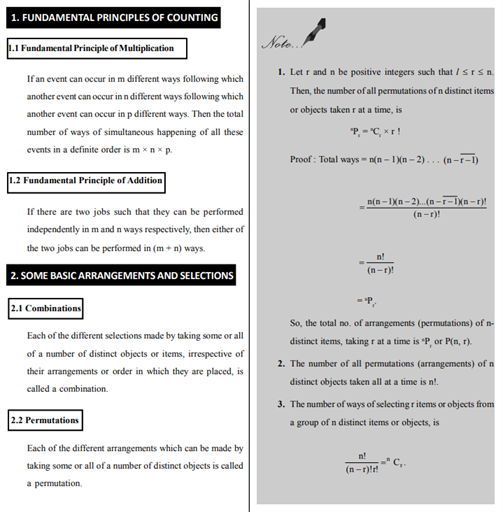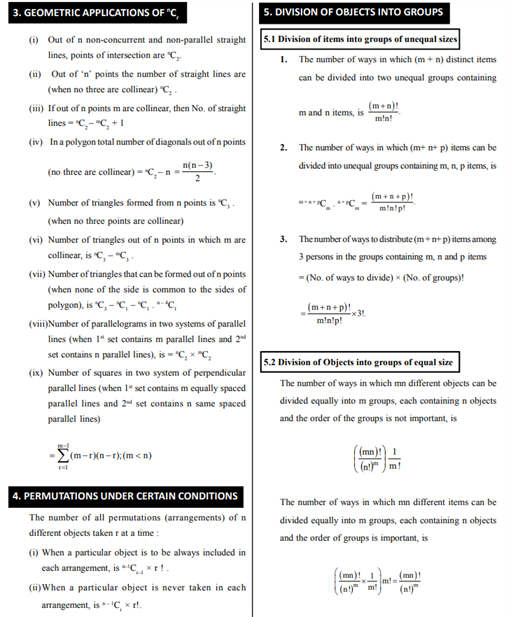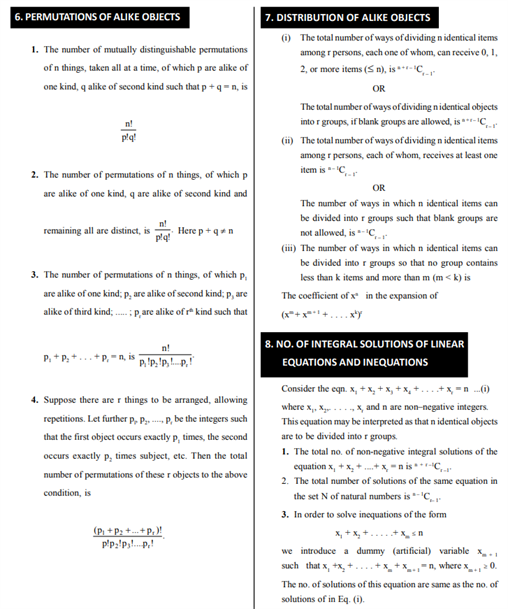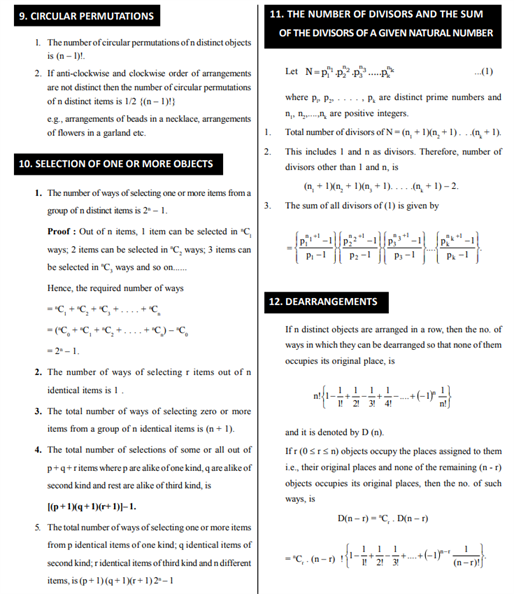# Revision Notes For CBSE Class 11 Maths Chapter 7 Permutations and Combinations

Here the class 11 maths revision notes chapter 7 Permutations and Combinations is provided. These notes will be very helpful to students who can find all the important concepts related to this chapter. For understanding, students will find the meaning of permutation below:

• The fundamental principle of counting (FPC)

Let’s suppose from place A, there are m ways to reach a place B and n ways to reach

place C.

Then by FPC, there are mn ways to go from station A to station C

• The number of permutations of n different things taken r at a time, where

repetition is not allowed is given by n

Pr = n(n-1)(n-2)…….(n-r+1)

where 0<r≤n.

They will get to understand the geometric applications of nCr and along with that, they will also see the division of objects into groups, permutations of alike objects. These notes even contain topics like dearrangement, circular permutations etc.. Practicing from these notes will give a deep understanding of this subject,

Get the class 11 maths revision notes Chapter 7 Permutations and Combinations here.